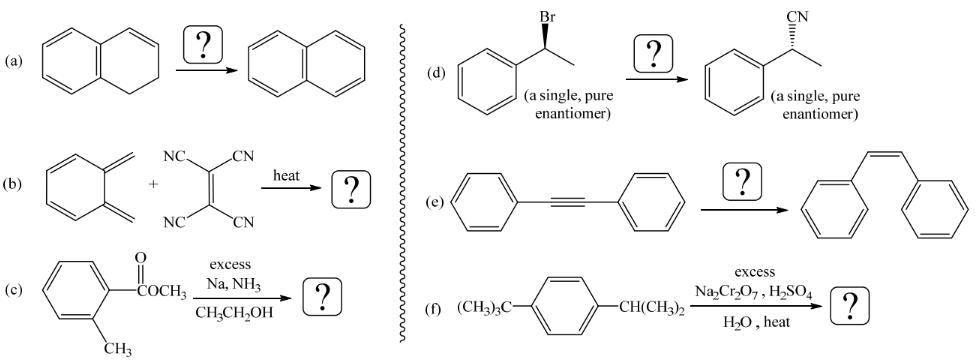# Problem: For each of the following, supply a structural formula for the major organic product(s) of the final reaction step when the product(s) is(are) not given; if no reaction occurs, write N.R. If the major product is a mixture (for example, enantiomers or stereoisomers), give a structural formula for each of the isomers in the mixture. Provide the following when a product is given. If an organic reactant is missing, supply a structural formula; if an inorganic reactant (reagent) or catalyst is missing, simply give a formula. If heat and/or light is needed, be sure to indicate it appropriately if these conditions are missing. If more than one reaction is needed, be sure to label each step numerically (1. ... 2. ..., etc.). Give the best possible answers. Be sure to show stereoisomers properly when necessary.

###### Problem Details

For each of the following, supply a structural formula for the major organic product(s) of the final reaction step when the product(s) is(are) not given; if no reaction occurs, write N.R. If the major product is a mixture (for example, enantiomers or stereoisomers), give a structural formula for each of the isomers in the mixture. Provide the following when a product is given. If an organic reactant is missing, supply a structural formula; if an inorganic reactant (reagent) or catalyst is missing, simply give a formula. If heat and/or light is needed, be sure to indicate it appropriately if these conditions are missing. If more than one reaction is needed, be sure to label each step numerically (1. ... 2. ..., etc.). Give the best possible answers. Be sure to show stereoisomers properly when necessary.